Blog

Tutorial

2021年5月21日

3D图形学中的矩阵变换（一）

二. 变换矩阵

1. 缩放矩阵（scale）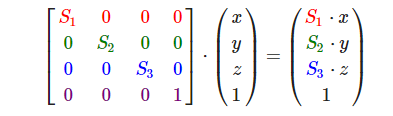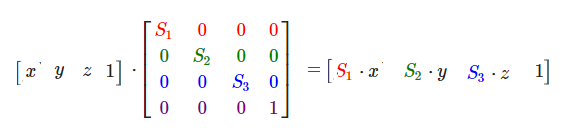2.平移矩阵（translation）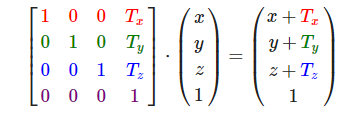3. 旋转矩阵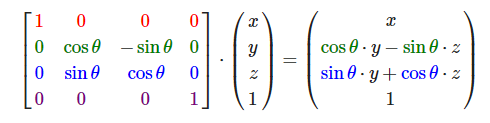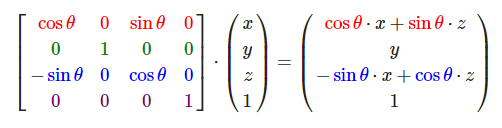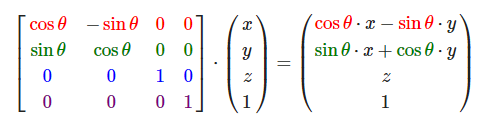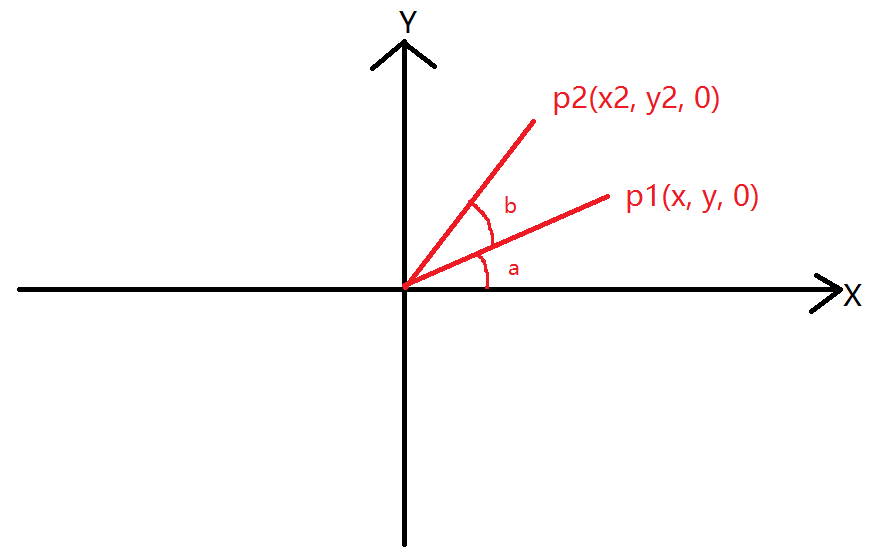// 经过旋转后向量的长度不变为L(原点到p1和p2的距离相同)
// 由三角函数得到sin(a + b), cos(a + b)的值
cos(a + b) = x2 / L;
sin(a + b) = y2 / L;

// 展开sin(a + b)和cos(a + b)
cos(a) * cos(b) - sin(a) * sin(b) = x2 / L;
sin(a) * cos(b) + cos(a) * sin(b) = y2 / L;

// 用x和y表示cos(a)和sin(a)
x / L * cos(b) - y / L * sin(b) = x2 / L;
y / L * cos(b) + x / L * sin(b) = y2 / L;

// 等式两边同时乘以L
x * cos(b) - y * sin(b) = x2;
y * cos(b) + x * sin(b) = y2;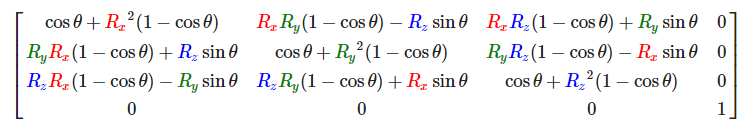first，我们可以将绕任意轴旋转转换成绕x，y，z轴旋转。所以先将轴(Rx, Ry, Rz)转换成某一个轴，例如转换成z轴。如图将轴绕Y轴旋转θ角度至yz平面。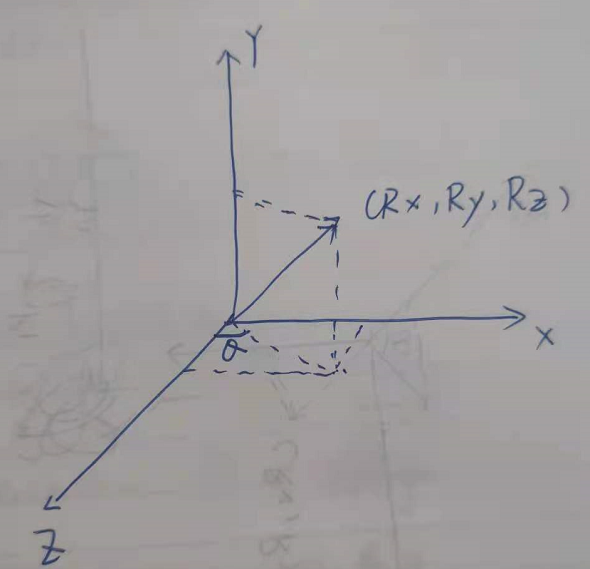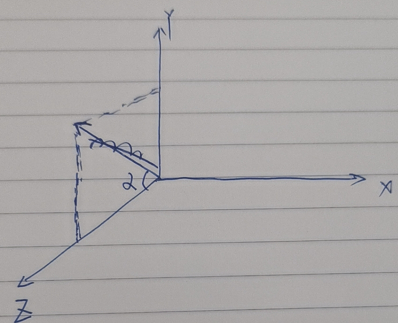（完）。